Quantum Mechanical Operators

The quantum mechanical methods, illustrated previously by the Schrödinger equation, are extended by the use of operators.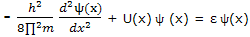Or, with h for h/(2∏), as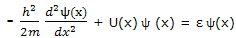For each of the set of functions that satisfies this equation, the quantity ε is the energy of the particle in the state corresponding to that solution function.

This equation, with which the energy corresponding to each allowed state is calculated, is just one of a number of equations that can be set up to calculate properties of quantum mechanical systems. All these expressions can be looked on as operator equations. Equation can be displayed to show this feature by writing it as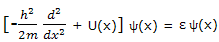This expression in the square brackets is an example of an operator. This particular operator is one dimensional Hamiltonian operator. It or its two-or three-dimensional counterparts are given the symbol H. with this notation, equation can be written as

H ψ = ε ψ

Earlier  we looked for functions that solved the Schrodinger equation, such as acted on by the operator H, give back a constant times the function. In this equation is the energy corresponding to that function. Functions that satisfy equations such as are known as eigenfunctoins, and the values of the constant, such as ε of equation are eigenvalues.

The energies of a system are identified as the eigenvalues for the Hamiltonian operator. Any other observable quantity has its own operator. The operator approach is therefore quite general. When an operator from an observable quantity operates on the wave function for the system and gives a result which is constant times the wave function, that constant is the value of the observable quantity.

Normalization: wave functions can be imaginary or complex, i.e. they can involve I = √-1. Let us now allow ψ to be such a function. Its complex conjucate, obtained by replacing I wherever it appears by -i, is denoted ψ *. A complex ψ is normalized if

∫ ψ * ψ d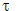= 1

Example: normalize the wave functions for a particle on a line given as ψ = (const) sin (n∏x/a).

Solution: a wave function in one dimension is normalized if ∫ ψ * ψ dx= 1. Here we require that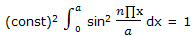The integral can be simplified by introducing y = n∏x/a, so that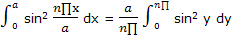Now the integration result given in integral tables can be used to obtain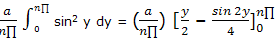= (a/(n∏)) ((n∏)/2)

= a/2

It follows that (const) = (2/a)1/2 and that the normalized wave function is

ψ = (2/a)1/2 sin n∏x/a

Example: use the normalized wave function expression ψ = (2/a)1/2 sin (n∏x/a) for a particle-on-a-line and the position operator to obtain the expectation value for the position of a particle on a line segment.

Solution: the position operator is the x coordinate and the expectation value is given by equation here we have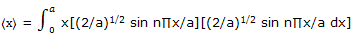Substitution of y = n∏x/a converts this to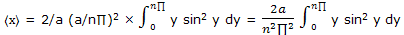Use of the integration result from tables of integrals then gives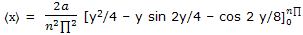= 2a/(n2 ∏2 ) (n22/4)

= a/2

We have come, by this formal procedure, to the result that the average, or expectation, value for the position of a particle on a line segment is at the middle of the segment. This result is apparent from symmetry of the wave functions.

#### Related Questions in Chemistry

• ##### Q :Mole fraction of solute The mole

The mole fraction of the solute in 1 molal aqueous solution is: (a) 0.027 (b) 0.036 (c) 0.018 (d) 0.009What is the correct answer.

• ##### Q :Crystals of covalent compounds Crystals

Crystals of the covalent compounds always contain:(i) Atoms as their structural units  (ii) Molecules as structural units  (iii) Ions held altogether by electrostatic forces (iv) High melting pointsAnswer: (i)

• ##### Q :Units of Measurement Unit of

Unit of measurement- These are also some systems for units:      (1) C.G.S.

• ##### Q :What is Distillation Separation by

Separation by distillation can be described with a boiling point diagram. The important process of distillation can now be investigated. From the boiling point diagram one can see that if a small amount of vapour were removed from a liquid of composit

• ##### Q :What is heat capacity and how to

The temperature reliance of internal energy and enthalpy depends on the heat capacities at constant volume and constant pressure. The internal energy and enthalpy of chemical systems and the energy changes that accompany chemical reactions depend on the

• ##### Q :Relative lowering of the vapour pressure

Choose the right answer from following.The relative lowering of the vapour pressure is equal to the ratio between the number of: (a) Solute moleules and solvent molecules (b) Solute molecules and the total molecules in the solution (c) Solvent molecules and the tota

• ##### Q :Coordination compounds discuss the

discuss the practical uses of coordination compounds, give reactions involves and explain whats happening in the process

• ##### Q :Production of alcoholic drinks give all

give all physical aspects in the production of alcohol

• ##### Q :Colligative property associated question

Give me answer of this question. Which of the following is not a colligative property : (a)Optical activity (b)Elevation in boiling point (c)Osmotic pressure (d)Lowering of vapour pressure

• ##### Q :Normality how 0.5N HCL is prepared for

how 0.5N HCL is prepared for 10 littre solution